# Samacheer Kalvi Books: Tamilnadu State Board Text Books Solutions

## Samacheer Kalvi 11th Computer Application Chapter 7 Spreadsheets Basics (OpenOffice Calc) Notes PDF Download: Tamil Nadu STD 11th Computer Application Chapter 7 Spreadsheets Basics (OpenOffice Calc) NotesSamacheer Kalvi 11th Computer Application Chapter 7 Spreadsheets Basics (OpenOffice Calc) Notes PDF Download: Tamil Nadu STD 11th Computer Application Chapter 7 Spreadsheets Basics (OpenOffice Calc) Notes

We bring to you specially curated Samacheer Kalvi 11th Computer Application Chapter 7 Spreadsheets Basics (OpenOffice Calc) Notes PDF which have been prepared by our subject experts after carefully following the trend of the exam in the last few years. The notes will not only serve for revision purposes, but also will have several cuts and easy methods to go about a difficult problem.

 Board Tamilnadu Board Study Material Notes Class Samacheer Kalvi 11th Computer Application Subject 11th Computer Application Chapter Chapter 7 Spreadsheets Basics (OpenOffice Calc) Format PDF Provider Samacheer Kalvi Books

## How to Download Samacheer Kalvi 11th Computer Application Chapter 7 Spreadsheets Basics (OpenOffice Calc) Notes PDFs?

2. Click on the Samacheer Kalvi 11th Computer Application Notes PDF.
3. Look for your preferred subject.
4. Now download the Samacheer Kalvi 11th Computer Application Chapter 7 Spreadsheets Basics (OpenOffice Calc) notes PDF.

Question 1.
Which is the first electronic spreadsheet?
(a) Excel
(b) Lotus 1-2-3
(c) VisiCalc
(d) OpenOffice Calc
(c) VisiCalc

Question 2.
Which of the following applications was the parent to OpenOffice Calc?
(a) VisiCalc
(b) LibreCalc
(c) Lotus 123
(d) StarOffice Calc
(b) LibreCalc

Question 3.
Grid of cells with a programmable calculator:
(b) Database
(c) Word processor
(d) Linux

Question 4.
A column heading in Calc as a:
(a) Number
(b) Symbol
(c) Date
(d) Alphabet
(d) Alphabet

Question 5.
Which key is used to move the cell pointer in the forward direction within the worksheet?
(a) Enter
(b) Tab
(c) Shift + Tab
(d) Delete
(b) Tab

Question 6.
A formula in Calc may begin with:
(a) =
(b) +
(c) –
(d) All the above
(d) All the above

Question 7.
What will be the result from the following formula? (Assume A1 = 5, B2 = 2)? + A1 B2:
(a) 7
(b) 25
(c) 10
(d) 52
(b) 25

Question 8.
What will be the result from the following expression? (AssumeH1 = 12, H2 = 12)? = H1<>H2:
(a) True
(b) False
(c) 24
(d) 1212
(b) False

Question 9.
Which of the following symbol is used to make a cell address as an absolute reference?
(a) +
(b) %
(c) &
(d) \$
(d) \$

Question 10.
Which of the following key combinations is used to increase the width of the current column?
(a) Alt + Right arrow
(b) Ctrl + Right arrow
(c) Alt + Left arrow
(d) Ctrl + Left arrow
(a) Alt + Right arrow

Question 1.
What are the types of toolbars available in OpenOffice Calc?
Under the menu bar, there are three toolbars available by default. They are:

1. Standard toolbar
2. Formatting toolbar
3. Formula bar

Question 2.
What is a Cell pointer?
Cell pointer is a rectangle box which can be moved around the worksheet. The cell in which the cell pointer is currently located is known as “Active cell”. When you type any content, it will appear in the active cell.

Question 3.
Write about the text operator in OpenOffice Calc?
In Calc, “&” is a text operator which is used to combine two or more texts as a single text. Joining two different texts is also known as “Text Concatenation”. An expression using the text operator has the following syntax:

Question 4.
Write the general syntax of constructing a formula in Calc?
General Syntax of constructing a formula is:
= cell referencel <operator> cell reference2 <operator>
Cell references are of two types

1. Relative cell reference
2. Absolute Cell reference.

If you refer cell addresses directly while constructing formulae, it is called as “Relative Cell reference”.

Question 5.
What are the keyboard shortcuts to cut, copy and paste?
Cut:
Cells you want to cut the formula using Ctrl + X.

Copy:
Copy the formula using Ctrl + C.

Paste:
Paste the copied formula using Ctrl + V.

Question 6.
Can you edit the contents of a cell? If yes, explain any one of the method of editing the cell content?
Yes, you can edit the contents of a cell in several distinct ways. Use this method convenient for you,

1. Double-click the cell you would like to edit. The cursor is placed where you double clicked.
2. Press the F2 key, the cursor is placed at the end of the text.
3. Click anywhere in the formula bar. The formula bar changes to have an entered and check mark.

Question 7.
What are the options available in “Insert Cells” dialog box?
The “Insert Cells” dialog box appear with four options.

1. Shift cells down
2. Shift cells right
3. Entire row
4. Entire Column

Question 8.
Match the following: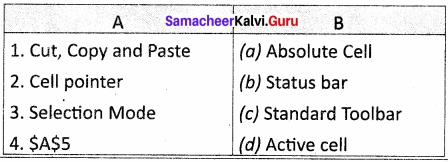1. (c)
2. (d)
3. (b)
4. (a)

Question 9.
Define the following
(i) Text Operator
(ii) Rows and Columns of spreadsheet.
(i) Text Operator:
In Calc, “&” is a text operator which is used to combine two or more texts as a single text. Joining two different texts is also known as “Text Concatenation”. An expression using the text operator has the following syntax:

(ii) Rows and Columns of spreadsheet: the formula bar contains the worksheet of work area which consist of grid cells The worksheet has number of rows and columns, where each column is labelled as A, B, C, D … AA, AB, AC … and the rows are numbered from 1,2,3 … OpenOffice Calc version 4.1.5 contains 1024 columns and 10,48,576 rows. Column heading starts from A and end with AMJ. In the case of Microsoft Excel 2016, there are 16,384 columns (A to XF(d) and 10,48,576 rows available.

Question 10.
Differentiate between Copy-Paste and Cut-Paste?
Copy – paste:

1. Select the cell or group of cell you want to copy, then using Ctrl + C (or) Edit → copy (or) copy icon.
2. Select the cells in which you want to paste, using Ctrl + V (or) Edit → paste (or) paste – icon.

Cut- paste:

1. Select the cell or group of cell you want to cut, then using Ctrl + X (or) Edit → cut (or) cut-icon.
2. Move the cell pointer to the cell in which you want to paste, using Ctrl + V (or) Edit → paste (or) paste-icon.

III. Explain in Brief

Question 1.
Write a short note on OpenOffice Calc?
OpenOffice.org (OOo), commonly known as OpenOffice, is the leading open-source office software suite for word processing, spreadsheets, presentations, graphics, database and more. It is available in many languages and works on all common computers. It stores all your data in an International open standard format and can also read and write files from other common office software package.

Question 2.
Write about inserting columns and rows in Calc?
Inserting columns:
In Calc, you can insert a new column anywhere in the worksheet.

Step 1:
Select the column where a new column should be inserted.

Step 2:
Right-click on the selected column name that you selected. A pop-up menu will appears.

Step 3:
click the “Insert Columns” option from the menu.

Now, a new column will be inserted to the left of the current column.
A new column can also be inserted using Insert → Columns command.

Inserting rows:
In Calc, you can insert a new row anywhere in the worksheet.

Step 1:
Select the row where a new row to be inserted.

Step 2:
Right-click on the row number a pop-up menu will appears.

Step 3:
click “Insert Rows” option from the menu.
Now, a new row will be inserted to above the current row.

Insert → Rows command is used to insert a new row.

Question 3.
Differentiate Deleting data using Backspace and Delete?

1. Backspace key is used to delete the character left of the insertion pointer of the cell.
2. Delete key is used to delete the character right of the insertion pointer of the cell.
3. This can be done while typing or after typing in the cell.

Question 4.
Write any three formatting options?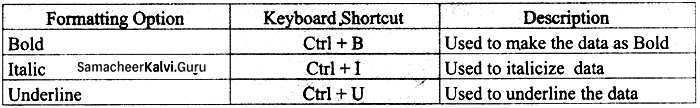Question 5.
In cell A1 = 34, A2 = 65, A3 = 89; write the formula to find the average?
To find the average, using anyone of the following ways.
Typing in the formula bar

1. = (A1+A2+A3)/3 (or)
2. = AVERAGE (A1; A3)

IV. Explain in detail

Question 1.
Explain about changing the column width in Calc?
Changing the column width in Calc in different ways available.
(i) Using mouse to change the column width. Keep the mouse button pressed and drag left or right in order to change the width of the column.

(ii) Using the dialog to change the column width. Click to choose Format → Column → Width, then enter the column width in the dialog box, which appears on the screen, then click OK button. Now that you want coloumn width increased.

Question 2.
Write the steps to generate the following series. 5, 10, 20 ……………….. 2560?

1. Select Edit → Fill → Series option is used to generate different set of series. Now fill series dialog box appeared.
2. Down / right / up / left, select the cell direction in the dialog box.
3. Choose growth series type, this type can generate multiplication series. This is suitable for given series.
4. Initial value of the series should be typed in the start value text box. That is 5.
5. End value of the series should be type in the end value text box, that is 2560.

Increment value between the first and second value of your series. So, the next value (third value) of the series will be generated based on this value. If you want to generate a decreasing order series, negative value should be specified an on increment value.

Question 3.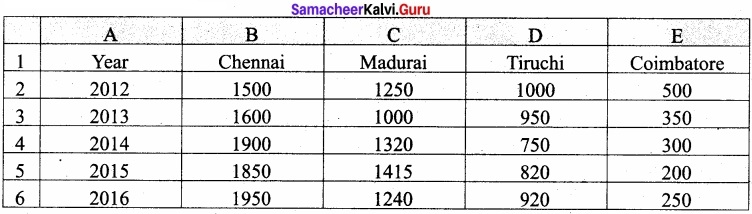Above table shows the sales figures for “Air Cooler” sold in four major cities of Tamilnadu from the year 2012 to 2016. Based on this data, write the formula to calculate the following.
(i) Total sales in the year 2015.
= SUM (B5 : E5) (or)
= B5 + C5 + D5 + E5

(ii) Total sales in Coimbatore from 2012 to 2016.
= SUM (E2 : E6) (or)
= E2 + E3 + E4 + E5 + E6

(iii) Total sales in Madurai and Tiruchi during 2015 and 2016.
= SUM (C5 : C6 ~ D5 : D6)

(iv) Average sales in Chennai from 2012 to 2016.
– AVERAGE (B2 ; B6) (or)
= (B2 + B3 + B4 + B5 + B6)/5

(v) In 2016, how many “Air Coolers” are sold in Chennai compared to Coimbatore?
= SUM IF (B2 : B6, > = , E2 : E6, “CHENNAI”, “COIMBATORE”)

Question 1.
……………………. is a very useful office automation tool for a tabular form.
(a) Word
(b) Calc
(d) Database

Question 2.
Which name of the spreadsheet software is Microsoft Corporation introduced?
(a) Lous 1-2-3
(b) Excel
(c) StarCalc
(d) VisiCalc
(b) Excel

Question 3.
The father of the spreadsheet is called:
(a) Charles Babbage
(b) Daniel Singer Dan Bricklin
(c) Stephen Hawking
(d) Bill Gates
(b) Daniel Singer Dan Bricklin

Question 4.
The popular open source spreadsheet software presently maintained by Apache foundation:
(a) VisiCalc
(b) OpenOffice Calc
(c) Excel
(d) StarCalc
(b) OpenOffice Calc

Question 5.
(a) Autosum
(b) Autofill
(c) Function
(d) Chart
(a) Autosum

Question 6.
Which feature guides you to work effectively while you work by displaying various helpful tips and techniques?
(a) Charts
(b) Wizard
(c) Function
(d) Autosum
(b) Wizard

Question 7.
Which feature can be used to create formulas to complex calculation?
(a) Charts
(b) Wizard
(c) Function
(d) Autosum
(c) Function

Question 8.
The main area of the OpenOffice code Calc window is called:
(a) Work area
(b) cell
(c) box
(d) function bar
(a) Work area

Question 9.
How many worksheet available in the spreadsheet by default?
(a) 1
(b) 2
(c) 3
(d) 4
(c) 3

Question 10.
Which menu contains the commands which are used to modify the environment of Calc?
(a) View
(b) Insert
(c) Tools
(d) Help
(a) View

Question 11.
Which menu lists inbuilt help features available with OpenOffice?
(a) View
(b) Insert
(c) Tools
(d) Help
(d) Help

Question 12.
The bar, which is used a very important elements in a spreadsheet:
(a) Formula bar
(b) Formatting toolbar
(c) Standard bar
(d) Scrolling bar
(a) Formula bar

Question 13.
Which box is used to show the contents of the current cell and shows what you typed in a cell?
(b) Name box
(c) Input line
(d) None of the above
(c) Input line

Question 14.
Which button is used to move the screen the relative distance?
(a) Scroll buttons
(b) Vertical scroll bar
(c) Horizontal scroll bar
(d) None of the above
(a) Scroll buttons

Question 15.
The intersection of every row and column makes a box, which is called:
(a) cell
(b) row
(c) column
(d) table
(d) table

Question 16.
The combination of column headings and row number:
(a) row number
(d) table

Question 17.
A rectangle element which can be moved around the worksheet:
(a) cell
(c) cell pointer
(d) active cell
(c) cell pointer

Question 18.
The cell in which the cell pointer is currently located is known as:
(a) cell
(c) cell pointer
(d) active cell
(d) active cell

Question 19.
How many navigation buttons are used to move the different worksheet?
(a) 1
(b) 2
(c) 3
(d) 4
(d) 4

Question 20.
At the bottom of the sheet tabs and horizontal scrolling bar is called:
(a) status bar
(b) formula bar
(c) standard bar
(d) scroll bar
(a) status bar

Question 21.
Which is shows current serial number of the sheet / total number of sheets available?
(a) Sheet count
(b) Page style
(c) Selection mode
(d) Zoom
(a) Sheet count

Question 22.
How many modes shows the selection mode of the current sheet?
(a) 1
(b) 2
(c) 3
(d) 4
(c) 3

Question 23.
When you open a new spreadsheet, the cell pointer is located in cell A1. So the cell A1 is known as:
(a) Cell pointer
(b) Home cell
(c) Bottom cell
(d) Direct cell
(b) Home cell

Question 24.
Which keys are used to move the cell pointer anywhere in the worksheet?
(a) Shift key
(b) Tab key
(c) Direction keys
(d) Home key
(c) Direction keys

Question 25.
How many direction keys are available to move the cell pointer in the worksheet?
(a) 1
(b) 2
(c) 3
(d) 4
(d) 4

Question 26.
How many data types are generally classified using spreadsheet?
(a) 2
(b) 3
(c) 4
(d) 5
(d) 5

Question 27.
In generally, the data can be entered in the cell, the default aligned is:
(a) left
(b) right
(c) center
(d) justify
(a) left

Question 28.
The Indian data format in the system:
(a) DD / MM / YYYY
(b) YYYY / MM / DD
(c) MM / DD / YYYY
(d) None of these
(a) DD / MM / YYYY

Question 29.
Combination of values, operators and cell references is called as:
(a) Data
(b) Operators
(c) Expression
(d) Reference
(c) Expression

Question 30.
How many operators can support the OpenOffice Calc?
(a) 1
(b) 2
(c) 3
(d) 4
(d) 4

Question 31.
Relational operators are also known as:
(a) Comparative operator
(b) Arithmetic operator
(c) Logical operator
(d) Boolean operator
(a) Comparative operator

Question 32.
A continuous group of cell is called as:
(a) range
(b) reference
(c) relational
(d) text
(a) range

Question 33.
How many types of reference operators are used in the spreadsheet?
(a) 1
(b) 2
(c) 3
(d) 4
(c) 3

Question 34.
Which is the range reference operator using worksheet?
(a) ,
(b) ;
(c) :
(d) –
(c) :

Question 35.
Which is the reference concatenation operator used in calc?
(a) ,
(b) ;
(c) ~
(d) :
(c) ~

Question 36.
Which is the Intersection Operator using OpenOffice calc?
(a),
(b) \
(c) ~
(d) !
(d) !

Question 37.
Which of the following operator is used as text operator?
(a) \
(b) ~
(c) &
(d) :
(c) &

Question 38.
How many types of cell reference are used in the worksheet?
(a) 1
(b) 2
(c) 3
(d) 4
(b) 2

Question 39.
The OpenOffice calc, spreadsheets are stored the default extension file name is:
(a) .xls
(b) .ods
(c) .txt
(d) .doc
(b) .ods

Question 40.
The extension file name of the animated image is:
(a) .gif
(b) .ods
(c) .jpg
(d) .mp3
(a) .gif

Question 41.
Which of the following combination key can be used as close the document?
(a) Ctrl + W
(b) Ctrl + C
(c) Ctrl + E
(d) Ctrl + L
(a) Ctrl + W

Question 42.
If you are not willing to save the file, then click:
(a) open
(c) ok
(d) save

Question 43.
The process of copy and paste can be replaced by a click and drag it is called as:
(a) autofill
(b) copy the cell
(c) move the cell
(d) delete the cell
(a) autofill

Question 44.
How many options having insert cells dialog box?
(a) 1
(b) 2
(c) 3
(d) 4
(d) 4

Question 45.
The user gives additional effect to the text in a cell is known as:
(a) formatting
(b) deleting
(c) copying
(d) moving
(a) formatting

Question 46.
Which of the shortcut key is to align the center the data within the cell?
(a) Ctrl + C
(b) Ctrl + E
(c) Ctrl + B
(d) Ctrl +1
(b) Ctrl + E

Question 47.
Which of the following shortcut key is used to visually change the format of a numeric content in currency symbol?
(a) Ctrl + Shift + 4
(b) Ctrl + Shift + 5
(c) Ctrl + Shift + 6
(d) Ctrl + Shift + 3
(a) Ctrl + Shift + 4

Question 48.
How many types are there to inserting functions into worksheet?
(a) 1
(b) 2
(c) 3
(d) 4
(b) 2

Question 49.
Which operator can be used in begins a function?
(a) +
(b) –
(c) =
(d) i
(c) =

Question 50.
Which combination of shortcut key can be involved in function wizard?
(a) Ctrl + FI
(b) Ctrl + F2
(c) Ctrl + F3
(d) Ctrl + F4
(b) Ctrl + F2

Question 51.
How many types of tabs are used in function wizard?
(a) 1
(b) 2
(c) 3
(d) 4
(b) 2

Question 52.
How many types function are categorized?
(a) 9
(b) 10
(c) 11
(d) 12
(c) 11

Question 53.
The OpenOffice Calc, arguments are separated by:
(a) ,
(b) –
(c) :
(d) .
(b) –

Question 54.
The one of the most important feature of spreadsheet is the ability to create that based on numeric data is:
(a) charts
(b) data
(c) series
(d) range
(a) charts

Question 55.
The chart should be created in the specify the range of data is called:
(a) charts
(b) data range
(c) series
(d) format
(b) data range

Question 56.
Which one of the method is used to re-arrange the items in ascending or descending order based on alphabets?
(a) Filtering
(b) Sorting
(c) Range
(d) Series
(b) Sorting

Question 57.
Which method are used to show only the selected portion of data from a large size database?
(a) Sorting
(b) Filters
(c) Series
(d) Data range
(b) Filters

Question 58.
How many types of filters are available?
(a) 1
(b) 2
(c) 3
(d) 4
(c) 3

Question 59.
The formatting aspect of the data tools lies in the virtualization of those data for easy understanding of the user that can you specify:
(a) conditional formatting
(b) filter
(c) sorting
(d) None of these
(a) conditional formatting

Question 1.
Name the some popular spreadsheet software?
VisiCalc, Lotus 1-2-3, StartCalc, MS-Excel, OpenOffice Calc etc.,

Question 2.
How to create a new worksheet in OpenOffice Calc?
A new spreadsheet can be created from various methods. From windows, select Start → All Programs → OpenOffice → OpenOffice Calc.
Double click on “OpenOffice” icon the desktop.

Question 3.
The outline of the window is very similar to other application windows of OpenOffice. The main area of the Calc window is called as “Work area” or “Worksheet”.
A worksheet is a grid of cells with a programmable calculator attached to each cell.

Question 4.
What is a cell and cell address?
Intersection of each row and column makes a box which is called as “Cell”. Each cell has its unique address. Cell address is the combination of column heading and row number.

Question 5.
How will you working with data in a worksheet?
When you open a new spreadsheet, the cell pointer is located in cell Al. So, the Cell A1 is known as “Home Cell”. Cell pointer can be moved anywhere in the spreadsheet using the • direction keys.

Question 6.
What is the uses of direction keys?
“Tab key” is used to move the cell pointer towards the right side or forward direction. “Shift+Tab” is used to move backward i.e. from right to left in a row. “Enter” key is also used to move the cell pointer. Enter moves the cell pointer to a cell below the current cell i.e. downwards. Four “direction keys” are used to move the cell pointer anywhere in the worksheet.

Question 7.
How can you entering formula in the worksheet?
In Calc, you can enter formulas in two methods, either directly into the cell or at the input line. Formula in Calc may start with equal (=) or plus (+) or minus (-) sign followed by a combination of values, operators and cell references. But, as a general , practice, all formulas should start with an equal sign. If any formula starts with a + or -, the values will be considered as positive or negative respectively.

Question 8.
What is an Expression?
Operators are symbols for doing some mathematical, statistical and logical calculations, combination of values, operators and cell references is called as “Expression”.

Question 9.
What are the variety of operators in Calc?

1. Arithmetic Operators
2. Relational Operators
3. Reference Operators
4. Text Operators.

Question 10.
What is meant by relational operators?
Relational operators are symbols used for comparing two values such as greater than, less than, equal to etc. The relational operators are also called as “Comparative operators”. These operators return either a True or a False.
List of Relational operators:
> – greater than
< – less than
> = – greater than equal to
< = – less than equal to
= – equal to
<> – not equal to

Question 11.
What is called range? Give its types?
Reference operators are used to refer cell ranges. A continuous group of cells is called as “Range”. There are three types of reference operators that are used to refer cells in Calc. They are –

1. Range Reference Operator
2. Range Concatenation
3. Intersection Operator

Question 12.
What is a Range reference operator?
Colon (:) is the range reference operator. It is used to group a range of cells. An expression using a range operator has the following syntax: reference left : reference right.

Where reference left is the starting cell address of a linear group of cells or upper left comer address of a rectangular group of cells. Reference right may be a last cell address of a linear group or lower right comer address of a rectangular group of cells.

Question 13.
What is reference concatenation operator and write the syntax with example?
Concatenation means joining together. Tilde (~) symbol is used as a concatenation operator in Calc. An expression using a concatenation operator has the following syntax: reference left ~ reference right.

Eg: If you want to find the sum of the values from Alto A6 and C3 to F3.
The formula is = SUM (A1 : A6 ~ C3 : F3)

Question 14.
What is a intersection operator and write the syntax?
Intersection operator is used to join two set of groups. It is very similar to Range concatenation operator. The intersection operator is represented by an exclamation, reference left reference right.

Question 15.
What are the steps should follow to construction of formula?
To construct a formula, you should follow the steps below:

1. Cell pointer should be in the cell in which you want to display the result.
2. Formula should begin with an = sign.
3. In a formula, use only cell reference (cell addresses) instead of the actual values within the cells.
4. While constructing a formula, BODMAS rule should be kept in mind.

Question 16.
What is a Absolute cell reference and give the example?
(i) While writing a formula, if you use the \$ symbol in front of a column name and row number, it will become an “Absolute Cell reference”.

(ii) Examples of Absolute cell references:
Adding values of A1, B1, C1, D1 = \$A\$1 + \$B\$1 + \$C\$1 + \$D\$1
Subtract E4 from H3 = \$H\$3 – \$E\$4.

Question 17.
What is the help of file extension in the file name?
A file extension or file name extension helps to identify the type of file. Following table gives the file extension of commonly used files.

Question 18.
What is meant by Auto save?
The OpenOffice saves a file at regular intervals. This is called as “Auto Save” feature. The default time interval is 15 minutes. It can be reduced even to one minute. If any unexpected shutdown occurs, this feature will recover your file.

Question 19.
How can you Inserting multiple columns?
Step 1: Select the column where a new column should be inserted.
Step 2: Right-click on the selected column name that you selected. A pop-up menu appears. Step 3: Click the “Insert Columns” option from the menu.
Now, a new column will be inserted to the left of the current column.

Question 20.
How can you delete multiple columns or rows in worksheet?
Multiple columns or rows can be deleted at a time.

1. Select the required columns or rows for deletion.
2. Right-click on the selected columns or row.
3. Choose Delete Columns or Delete Rows from the pop-up menu or Edit → Delete Cells.

Question 21.
Write the syntax of direct insert method SUM function?
SUM is the most frequently used function to add a set of values.
The syntax of the SUM ( ) is
= SUM (range 1; range 2; range 3 ……….. range n)
If you want to know the sum of the values on A1, A2, A3, A4, A4 in A5, Place your cell pointer in A5 and directly type the formulae as follows.
= SUM (A1 : A4)

Question 22.
What are the points should be kept in mind while inserting a function?
While inserting a function the following points should be kept in mind.

1. A function should begin with an equal sign.
2. Use proper name of the function to be used.
3. Arguments should be given within the brackets as per the syntax. Each function has unique argument list.
4. Press, “Enter” key aftef typing the function.

Question 23.
Describe the chart?
One of the most important features of spreadsheet is the ability to create charts based on numeric data. The charts are used to present data in an easy manner. Creating charts is the key factor for the of success of spreadsheet. OpenOffice Calc provides a “chart wizard” to create and manipulate charts.

Question 24.
What are the types of chart wizard?
Chart wizard has 4 steps viz.

1. Chart type
2. Data Range
3. Data Series and
4. Chart Elements.

Question 25.
What is meant by sorting?
Sorting is the process of arranging data in ascending or descending order. There are two types of sorting in OpenOffice Calc. They are,

1. Simple Sorting
2. Multi Sorting
3. Sort by selection.

Question 26.
What is the use of filters with types?
Filter is a way of limiting the information that appears on screen. Filters are a feature for displaying and browsing a selected list or subset of data from a worksheet. The visible records satisfy the condition that the user sets. Those that do not satisfy the condition are only hidden, but not removed. OpenOffice Calc allows three types of filters. They are AutoFilter, Standard Filter and Advanced Filter.

Question 27.
How can you change the worksheet name?
Every sheet name can be renamed. To rename a sheet, just double-click on the sheet, which will show a small box. It shows the current name; delete or overwrite the existing name and type a new name; click OK button. New name will be displayed on the sheet.

Question 28.
What is the hierachy of operators using in arithmetic Calculation?
When arithmetic operators are used in a formula, Calc calculates the results using the rule of precedence followed in Mathematics. The order is:

1. Exponentiation ( A )
2. Negation (-)
3. Multiplication and Division ( *, /)
4. Addition and Subtraction (+, -)

Question 29.
What is the use of formatting text?
Formatting Data in a cell gives additional effect to the text. Additional effect includes changing the font style, font size, automatic wrapping, bold, underline, italic etc. The data in Calc can be formatted in several ways. Using formatting icons can be used.

Question 30.
What is save?
Technically saving is a process of transferring or shifting contents from primary memory. (RAM) to Secondary storage medium such as Hard disk, Pen drive, memory chip etc.

III. Explain in Brief

Question 1.
Write the important elements in the formula bar?
Formula bar:
This is a very important element in a spreadsheet. It contains Name box, Function Wizard, Sum button, Function button and Input line.

Name box:
It display the current cell address

Function Wizard:
It is used to insert function

Sum button:
It is used to quickly insert sum function

Input Line:
This is used to show the contents of the current cell. It always shows actually what you typed in a cell. It is also used to edit the contents.

Question 2.
What is the use of scrolling bar in the spreadsheet?
Spreadsheet window also has two sets of scrolling bars.

1. Vertical Scrollbar
2. Horizontal Scrollbar
3. Scroll Buttons

1. Vertical Scroll bar:
It is used to move the screen up and down.

2. Horizontal Scroll bar:
It is used move the screen left and right.

3. Scroll buttons:
Used to move the screen the relative distance.

Question 3.
What are the uses in the navigation buttons?
On the left of the sheet tab, four navigation buttons are used to move sheets if you have more number of worksheets.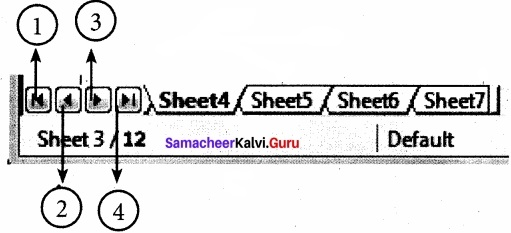1. Move to the First sheet
2. Move to the previous sheet
3. Move to Next sheet
4. Move to the Last sheet

Left comer of status bar shows the total count of sheets and the present active sheet number.
Eg: if the status bar shows sheet 3/12; 3 refers to the serial number of the current sheet and 12 refers to the total number of sheets available.

Question 4.
What are the different types of Data types?
Data are in different types. Data are made up of alphabets, numbers, Date and time is another data type even though it has numbers and symbols. In general, data types are classified as:
Alphabetic data type – consists of alphabets only.
Numeric data type – consists only numbers (whole number or fractional numbers)
Alphanumeric data types – consists of a combination of alphabets and numerals
Date data type – consists only dates
Time data type – consists only time

Question 5.
How will you save the worksheet?
The process of saving a worksheet is very similar to saving a document. Steps to save a worksheet are as follows:
Step 1: File → Save (or) Ctrl + S (or) Click “Save” icon on the standard tool bar.
Step 2: If the spreadsheet has not been saved previously, the Save As dialog box will appear.
Step 3: Type the name in “File Name” list box. OpenOffice Calc Spreadsheets are stored with extension .ods by default.
Step 4: Click ‘Save’ button.

Question 6.
How can you open an existing worksheet?
To reopen an existing worksheet, the File → Open command (or) “Open” icon (or) Ctrl + O can be used. An Open dialog will appears that is similar to “Save As” dialog box.
The name of the file to be opened can be chosen from the list or folder in which worksheet has been saved.

Question 7.
Write about power ( ) function?
POWER ( ) is a function which is used to calculate power of an exponent value of a number. This function is categorized as a Mathematical function. There are two inputs needed to find the power value of a number. They are, Base value and exponent value. For example, to find the value of 25 is the base value, 2 is the exponent value.
Hence, the syntax of POWER ( ) is
= POWER (Base ; Exponent)
Both Base and Exponent are arguments. In Calc, arguments are separated by a semicolon.

IV. Explain in detail

Question 1.
Write the any 5 features of OpenOffice Calc?
(i) Connecting with Excel:
Ability to open, edit, and save Microsoft Excel spreadsheets.

(ii) AutoSum:
Helps you to add the contents of a cluster of adjacent cells.

(iii) List AutoFill:
Automatically extends cell formatting when a new item is added to the end of a list.

(iv) AutoFill:
Allows you to quickly fill cells with repetitive or sequential data such as chronological dates or numbers, and repeated text. AutoFill can also be used to copy functions. You can also alter text and numbers with this feature.

(v) Charts:
helps you in presenting a graphical representation of your data in the form of Pie, Bar, Line charts and more. OpenOffice Calc provides a ‘Chart Wizard’ to create and manipulate charts.

(vi) Functions:
which can be used to create formula to perform complex calculations on data.

(vii) Database functions:
To arrange, store, and filter data.

Question 2.
Draw a OpenOffice Calc window and mark the parts?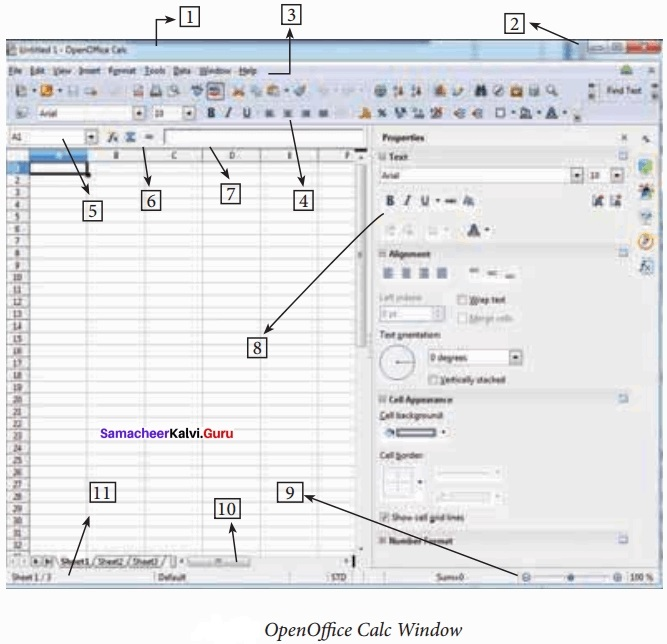1. Title Bar
2. Control Buttons
4. Tools Bar
5. Name Box / Address Box
6. Quick Function Wizard
7. Formula Bar / Input Line
8. Formatting Properties
9. Zoom
10. Scrollbar
11. Status Bar

Question 3.
Below the title bar is menu bar. Most of the menus are very similar to what you learn in OpenOffice Writer.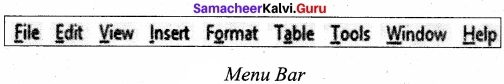(i) File:
Menu contains the commands of all file management tasks like, Create a new file, Open an existing file, Close the current file, Save a file, Save a file in another name, print file, Export file etc.

(ii) Edit:
Menu contains the editing commands like, cut, copy, paste, Undo, Redo, Fill etc., Most of the menu items are similar to Writer Edit menu. But, for Calc, some special editing options are available under this menu.

(iii) View:
Menu contains the commends which are used to modify the environment of calc.

(iv) Insert:
Menu contains commands for inserting various calc elements such as cells, columns, rows, functions, charts etc.,

(v) Format:
Menu contains the commands of various text and cell formatting features.

(vi) Tools:
Menu contains various tools and functions such as spell check, protect document, insert pictures, macros, etc.,

(vii) Data:
Menu contains the commands to manipulate data in a spreadsheet such as sort, filter, subtotal, validity etc.,

(viii) Window:
Menu shows display options such as New Window, Close Windows, Split and Freeze.

(ix) Help:
Menu lists in-built help features available with OpenOffice.

Question 4.
Explain the status bar in the worksheet?
Below of the sheet tabs and horizontal scrolling bar is “Status Bar”. It shows the current status of the worksheet.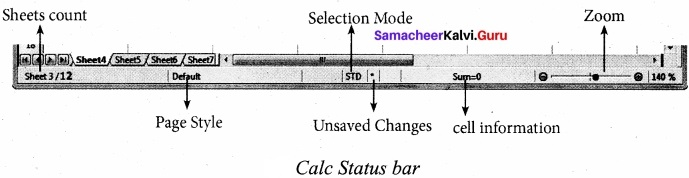(i) Sheets count:
Displays current serial number of the sheet / total number of sheets available.

(ii) Page Style:
Displays the page style of the current sheet. To make changes, just double¬click on “Default” and it will show you the “Page Style” dialog box, which is used to change the margin, orientation, paper size, inserting header, footer, border style etc.,

(iii) Selection Mode:
Displays the selection mode of the current sheet. There are three modes available to select the cells of a worksheet. They are, Standard (ST(d), Extend (EXT) and Add (AD (d).

(iv) Unsaved Changes:
An asterisk ( * ) symbol indicates the changes made in the worksheet that are not yet saved. If you saved your changes, it will disappear.

(v) Cell information:
Shows the sum of the values of selected cells.

(vi) Zoom slider:
used to increase or decrease the view scale (zoom). The default zooming scale is 140%.

Question 5.
Tabulate the Arithmetic operators with example?
Arithmetic operators are the symbols for performing simple arithmetic operations such as addition, subtraction, multiplication, division etc., These operators return a numerical result.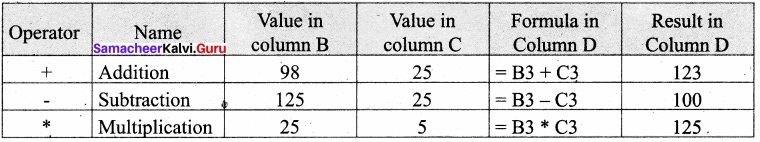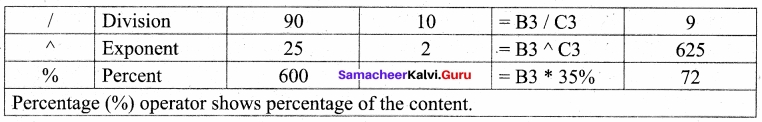Question 6.
Tabulate the Relational operators with example?
Relational operators are symbols used for comparing two values such as greater than, less than, equal to etc. So, the relational operators are also called as Comparative operators. These operators return either a True or a False.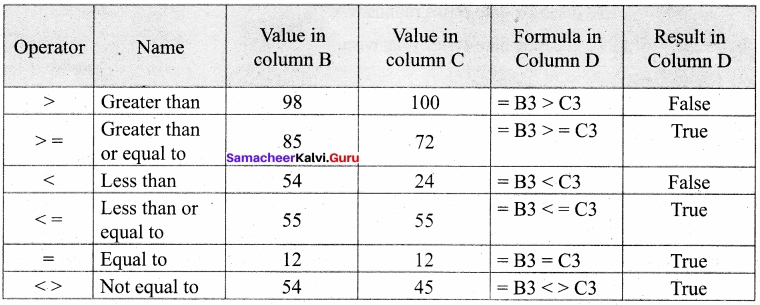Question 7.
How can you generating series in a worksheet?
Edit → Fill → Series Command is used to generate different set of series. Before using this feature, a set of cells should be selected. Using Fill Series feature, you can fill series of values at any direction. (Remember that, auto fill only fills either right or down).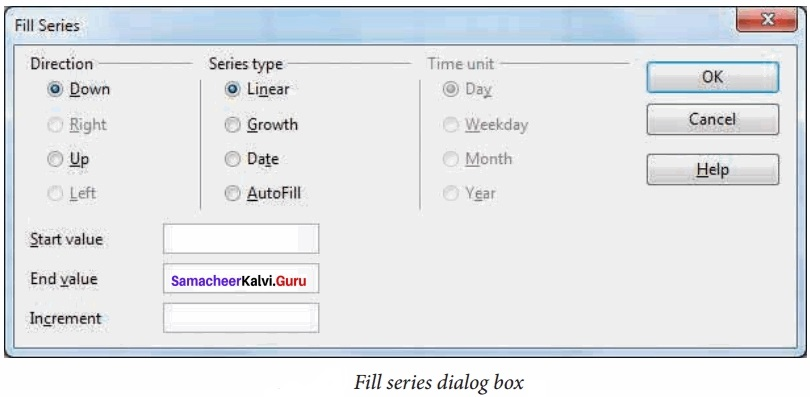Direction:
Down / Right / Up / Left (Selected cell direction will be default).

Series type:

Linear:
To generate addition series (2, 4, 6, 8, 10 )

Growth:
To generate multiplication series (2, 4, 8, 16, 32, 64 ………………)

Date:
To generate date series (when you select date as series type; time unit section gets enabled.)

AutoFill:
To generate a continuous series of values (1, 2, 3, ). When you select “AutoFill”, time unit section and End value, increment text boxes become disabled.

Time Unit:
(Enabled only when you select the series type as “Date”.) To generate date series day-wise To generate date series weekend-wise To generate date series month-wise

Year:
To generate date series year-wise

Start Value:
Initial value of the series should be typed

End Value:

1. End value of the series should be typed
2. If you fail to specify the end value, Series will be generated upto the selected cells.
3. If your selection is less than the specified end value, series will be generated only upto the selected cells.

Question 8.
Write the any five familiar file type of extension?
Familiar File Type:

1. Text Files
2. Microsoft Word Documents
3. OpenOffice Documents
4. Microsoft Excel
5. OpenOffice Calc
6. Microsoft PowerPoint

Extension:

1. .txt
2. .doc/.docx
3. .odt
4. .xls / .xlsx
5. .ods
6. ppt / .pptx

Question 9.
What are the types of Function categorised in a drop down list box?
Function Wizard has two tabs viz. Functions and Shortcuts. In Functions Tab, the list of categories is available in Category drop down list box. In Calc, the functions are categorized into 11 types. They are,

1. Database
2. Date and Time
3. Financial
4. Information
5. Logical
6. Mathematical
7. Array
8. Statistical
10. Text

Question 10.
How can you Insert and using power ( ) function in a worksheet?
Step 1:
In cell A2 type the base value 25.

Step 2:
In cell B2 type the exponent value 2.

Step 3:
Move the cell pointer to C2; in which you want display result.

Step 4:
Click (fx) icon from Formula bar (or) choose Insert —► Functions (or) Press Ctrl + F2.

Step 5:
Pull down category list box, Choose “Mathematical”.
All function under Mathematical category displayed in the “Functions” list box.

Step 6:
Scroll the “Functions” list box and select “POWER ( )”.
The function wizard shows description about the selected function on the right comer of the dialog box.

Step 7:
Click “Next” command button. Now, Function wizard appears.

Step 8:
Click on the cell which is contains the base value (A2).
Now, Minimized wizard shows the cell address you selected (A2).

Step 9:
Click “Maximize” button to display full wizard. Now, Function wizard appears.

Step 10:
Directly type the cell address which is contains exponent, in Exponent box; or repeat steps 7, 8 and 9.
The Formula box the displays syntax of the with input values and Result box displays the result value.

Step 11:
Result Function box at the top right comer is also shows the result of the function.
If the result is correct, click “OK” button, else click “Back” button, to display the previous page of this wizard.

Question 11.
How an you create a chart and write the steps?
The following steps is followed to create a chart for the given data.
Step 1:
Select Data: Select the data from A1 to F11.

Step 2:
To open the Chart Wizard: Click “Chart” icon from Standard toolbar (or) choose
Insert → Chart.

Step 3:
Selecting Chart type and shape: Select “Chart type” as Column and style as Normal
Check “3D look” and click “Cylinder shape”. Click “Next” button.
If the user wants change any other type or shape, click on the style image. Preview of the chart displayed on the backgroup of the chart wizard. So, you can view the chart at every stage of design.

Step 4:
Defining Data range:
In this case, the data has been selected earlier. So, the selected data range is displayed in “Data range” box.
Other settings are by default, “Next” to move step 5.

Step 5:

• “Data Series” list box shows all the columns to be included in the chart.
• Click on the “Total” in the data series box and click “Remove” button to remove the column.
• If you don’t want to add or remove any other column click “Next” button to move to the last step.

Step 6:
Adding Title, Subtitle, Name of X and Y axis:

• Type the title and subtitle of the chart in “Title” and “Subtitle” box.
• Type the name of the X and Y-axis in the respective boxes.
• The display layout textbox is selected to the right which is the default.
• Click “Finish” button.

Question 12.
What is a filter and explain its types?
Filter is a way of limiting the information that appears on screen. Filters are a feature for displaying and browsing a selected list (or) subset of data from a worksheet. The visible records satisfy the condition that the user sets. Those that do not satisfy the condition are only hidden, but not removed.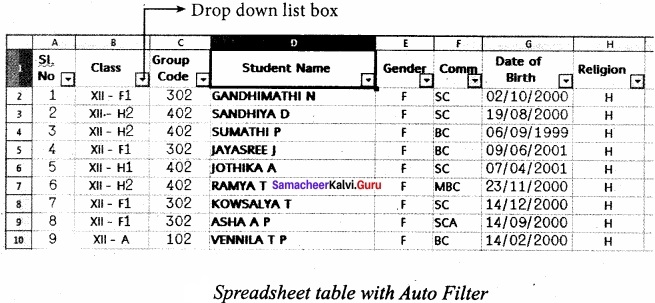OpenOffice Calc allows three types of filters. They are AutoFilter, Standard Filter and Advanced Filter.

(i) Auto Filter:
Auto Filter applies a drop-down list box to each field (columns) filled with similar data available in that field. Using the list box item, you can filter the data that matches the criteria of the data concerned.

Using Auto Filter:

• Click Auto Filter icon available on the “Standard tools bar” (or) Click Data → Filter → Auto Filter.
• The list box contains similar data in the fields.
• Each list box item will be considered as filter criteria.
• Select the data iterrf from the list box. Now, Calc shows only the records which are satisfy the selected criteria.

Removing Auto Filter:

• To remove auto filter, click “Auto filter” icon once again .
• The original table is displayed without filter.

(ii) Standard Filter:
Auto filter is used only for single criteria on a data, whereas the Standard filter is used for multiple critieria to filter.

Step 1:

1. Select Data → Filter → Standard Filter.
2. Now, the entire data is selected and “Standard Filter” dialog box dispalys.

Step 2:

1. Select the column heading from the “Field name” list box for first criteria.
2. Select conditional opeator such as >, <, = etc., from “Condition” list box.
3. Type or select the value of criteria in the “Value” box.

Step 3:

1. Select the one of the logical operator (And / Or) from “Operator” list box to fix second criteria.
2. Follow the step 2, for the next criteria.

Step 4:
Click “OK” to finish.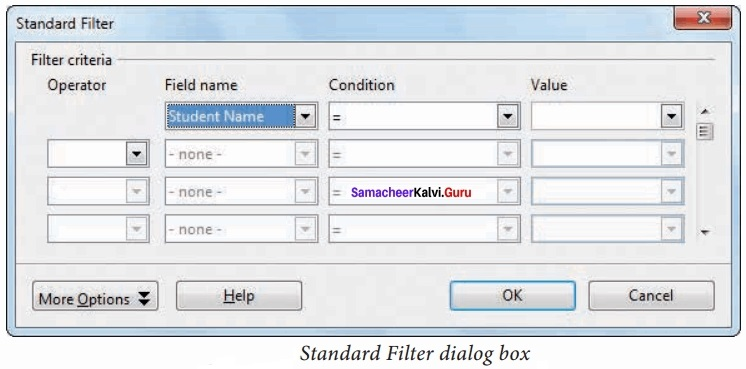Remove Standard Filter:
Select Data → Filter → Remove Filter.

## How to Prepare using Samacheer Kalvi 11th Computer Application Chapter 7 Spreadsheets Basics (OpenOffice Calc) Notes PDF?

Students must prepare for the upcoming exams from Samacheer Kalvi 11th Computer Application Chapter 7 Spreadsheets Basics (OpenOffice Calc) Notes PDF by following certain essential steps which are provided below.

• Use Samacheer Kalvi 11th Computer Application Chapter 7 Spreadsheets Basics (OpenOffice Calc) notes by paying attention to facts and ideas.
• Pay attention to the important topics
• Refer TN Board books as well as the books recommended.
• Correctly follow the notes to reduce the number of questions being answered in the exam incorrectly
• Highlight and explain the concepts in details.

## Frequently Asked Questions on Samacheer Kalvi 11th Computer Application Chapter 7 Spreadsheets Basics (OpenOffice Calc) Notes

#### How to use Samacheer Kalvi 11th Computer Application Chapter 7 Spreadsheets Basics (OpenOffice Calc) Notes for preparation??

Read TN Board thoroughly, make separate notes for points you forget, formulae, reactions, diagrams. Highlight important points in the book itself and make use of the space provided in the margin to jot down other important points on the same topic from different sources.

#### How to make notes for Samacheer Kalvi 11th Computer Application Chapter 7 Spreadsheets Basics (OpenOffice Calc) exam?

Read from hand-made notes prepared after understanding concepts, refrain from replicating from the textbook. Use highlighters for important points. Revise from these notes regularly and formulate your own tricks, shortcuts and mnemonics, mappings etc.
Share: